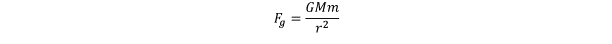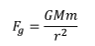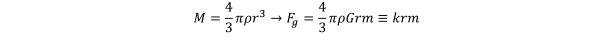# brand new

The pull of that mass gravitationally, Fg, is given by the classic equationNow add some text and hope it’s not overwritten – oops, it is overwritten. Kutools always converts equations to full-width images! Useless

Here I just used print screen, crop, copy, and paste – tedious!Here, m is the mass of our circling object or star, and G is the universal gravitational constant. Let’s express M in terms of the radius and the density of dark matter:Here, I gathered all the constants into a single constant, k.

The star is moving with a speed v (I call it speed because velocity is a vector, a speed in a specified direction. That direction is constantly changing as the star orbits, but, for a circular orbit we’re considering, the speed stays the same). The centrifugal force, Fc, is then given by another classic equation,